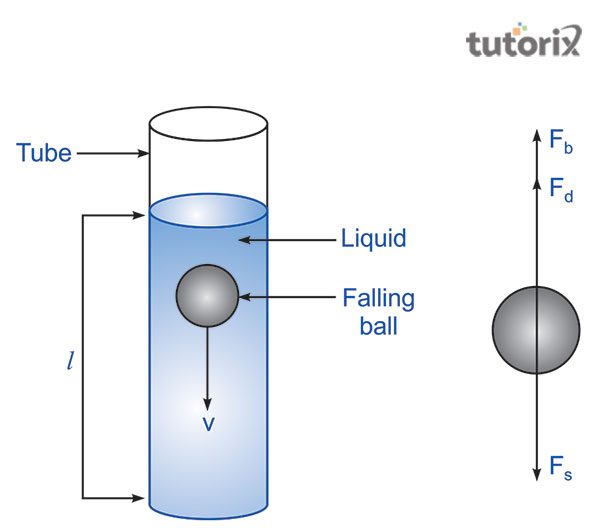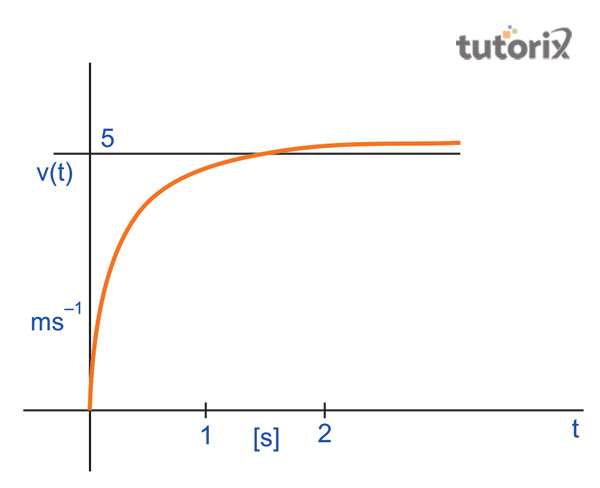# To determine the coefficient of viscosity of given viscous liquid by measuring terminal velocity of given spherical body

The measurement of the fluid or gas is defined as the viscosity of the respective gas or liquid. This specific concept helps to determine the fiction along with the movement of liquid. Experiments have stated that liquid that has a low viscosity can flow easily because while being in motion, the molecules of the liquid develop fiction. Based on these concepts, the tutorial will explain an experiment that helps to determine the coefficient viscosity of the liquid by measuring the terminal velocity of a given spherical object.

## Aim

The main of this experiment is to determine the coefficient viscosity of a given liquid that is viscous while measuring the terminal velocity of the given spherical body.

## Material required

In order to conduct this particular experiment to determine the aim of this experiment, several materials are required. The first required material is transparent viscous liquid which needs to be half a meter high (Iopscience, 2022). The glass cylinder needs to be 5centermeter broad along with millimetre graduations. Apart from these materials, the other required elements are clamp withstand, screw gauge, a steel ball, stopwatch and a thermometer.

## Theory and diagramFigure 1: Small sphere falling into a long glass cylindrical Jar

The theoretical formula that is followed in this experiment is the formula of terminal viscosity. The mathematical representation of this particular formula is V= 2r2(p-σ)g/9η. In this formula, the letter V is the representative of terminal viscosity and r represents the radius of the spherical body (Kong et al. 2019). σ, g, and p respectively represent liquid's density, acceleration duo gravity, and density of ball. η represents the coefficient of viscosity. In order to determine the coefficient of viscosity of the spherical body in the viscous liquid, a metal ball has been dropped in the liquid. The above figure represents the diagram of the falling of a spherical body in a jar that is filled with transparent viscous liquid.

## ProcedureFigure 2: Free body force diagram

In order to begin the experiments, the half meter high glass jar is required to be filled with transparent viscous liquid. At this time, one should docs on the visibility of the count on the jar that is placed vertically (Dietemann et al. 2020). The spring stopwatch needs to be attached to the glass jar that is required to e tightly clamped in order to identify the zero error and least count. The radius of the metal ball requires to be found prior to dropping the ball in the liquid. The ball is required to be fallen with accelerated viscosity till it reaches at least 1/3rd of the liquid’s height which helps to form the terminal velocity.

After gaining the terminal velocity, as the ball will reach the intended division, the stopwatch is required to be started. After the ball is observed to be reached the lowest division, the stopwatch is stopped in order to record the time that has been ten by the ball (Hagemeier, Thévenin & Richter, 2021). To get more readings of the occasion, the phenomenon is repeated 6 to 9 times. This is helpful for the reading the temperature of liquid long for recording observation

## Observation and resultFigure 3: Drag Forces in Fluids

The least count of the liquid is generally observed through this experiment and is measured by the vertical scale to 1mm. The least count of the attached stopwatch will be represented by the unit of seconds. The recorded zero hours also will be represented according to the unit of second. The Pitch of screw gauge that is represented by the letter p is determined to be equivalent to 1 mm where the number of divisions of the circular scale is equal to 100 (Jain, Tschisgale & Fröhlich, 2019).

Following this the zero error and the zero correction of screw gauge is represented as per the unit of mm. Based on the above figure and the distance fallen has been found and represented with the unit cm where the direction is determined with D1 and D2 and the time taken is respectively t1,t2,t3.

## Precautions

The main precaution that one is required to follow is that the taken liquid needs to be transparent and the used ball needs to be perfectly spherical. During the experiment, the count of velocity must be recorded only when it appears as a constant.

## Conclusion

The present tutorial has represented and explained an experiment based on the previously-mentioned aim of the respective experiments. In the first segment, the tutorial has explained the aim and the required materials for the experiment such as a cylindrical glass jar, a steel ball, transparent viscous liquid and thermometer. Along with this, the tutorial has further discussed the formal terminal viscosity which has been represented through a mathematical representation, V= 2r2 (p-σ) g/9η. After conducting the experiment, the result has been stated based on the observation. As a result, the coefficient viscosity of the liquid has been found with the CGS unit of temperature.

## FAQs

Q1. Why the liquid must be transparent?

In this experiment, the motion of the ball needs to be observed. Therefore, to see the motion of the ball, the liquid requires to be transparent.

Q2. Why hot water moves faster than cold water?

Through experiments, it has been noted that the viscosity of the hot water is smaller in comparison to the cold water. Therefore, the cold water moves slower than the hot water.

Q3. What is the unit of dynamic viscosity?

The SI unit of the dynamic viscosity is generally represented by the Greek letter μ, known by the name of Pascal-second. The value of Pascal-second second is considered to be equivalent to 1 kg m−1 s−1.

Q4. How does the viscosity of liquid and gases change?

The more temperature will be increased, the more the viscosity of the liquid will be reduced. On the other hand, the viscosity of the gasses will be increased as the temperature increases.